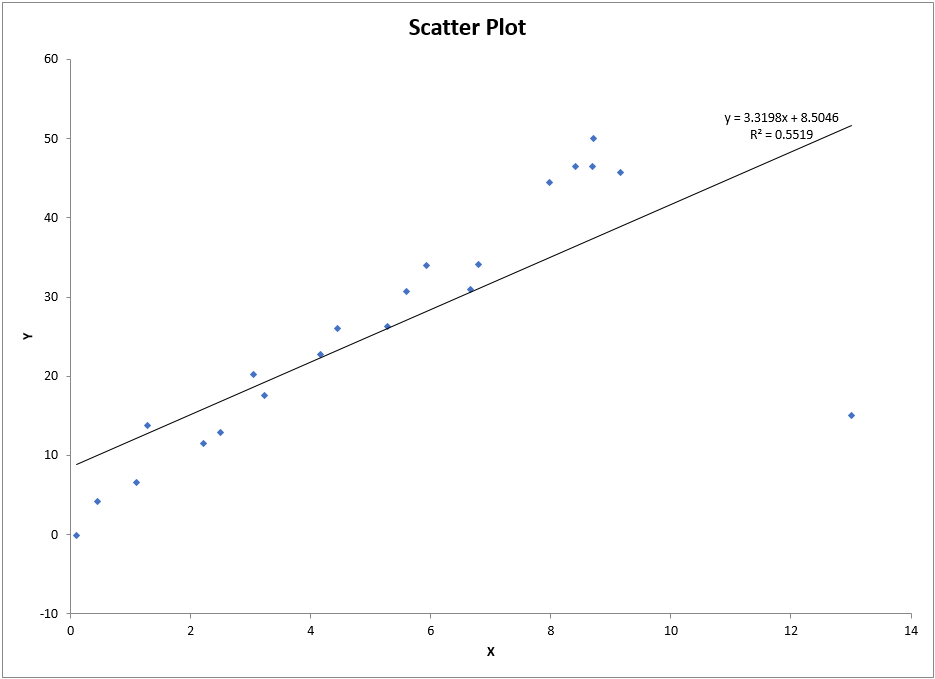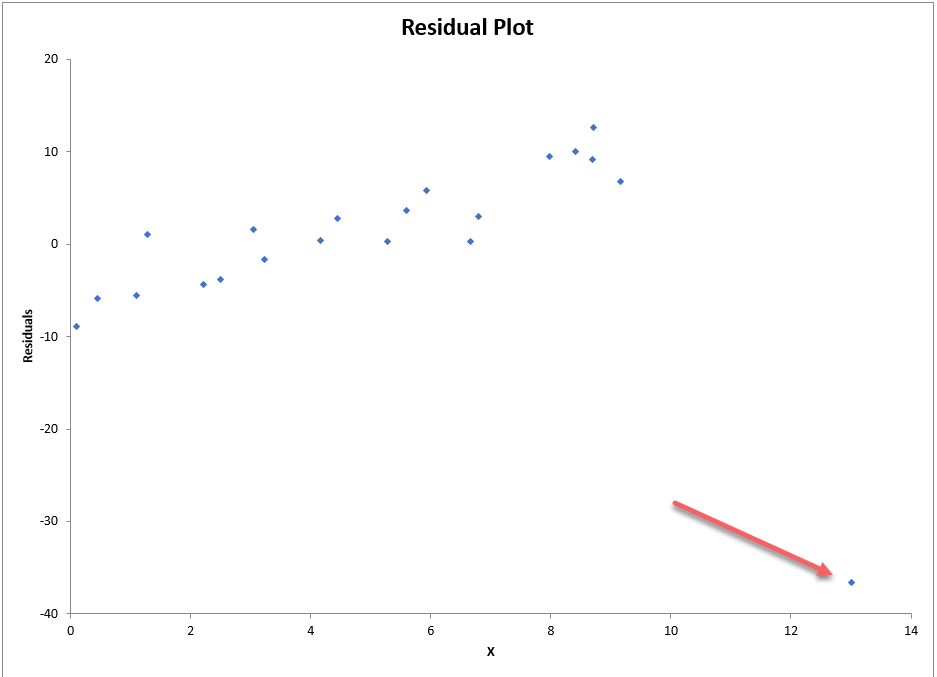# What to do about outliers?

The most recent assignment in my BUS 430 class was on simple linear regression. In some of the data sets, there are data points that seem to be inconsistent with the bulk of the data. One student called this to my attention and asked if he should just ignore those data points because they were “obviously a mistake.” His comment reminded me that in an earlier assignment, we had discussed briefly using a box plot software tool to identify outliers, but we had not discussed what to do about them.

When dealing with just two variables, it is quick and easy to make a scatter plot and inspect it for data points that do not follow the trend of the rest of the data. In the scatter plot below, we can see just such a data point in the lower right.But using a box plot does not identify this point as an outlier in either the x or y axis:Fortunately, most regression software allows us to find and plot the residuals – the differences between the actual values and the predicted values for each data point using the regression equation. The residual plot below clearly shows that the data point (red arrow) in question does not follow the trend of the others.So, based on the residuals, we can safely say the point is an outlier and we need to consider it further. The next question we should ask ourselves is: “Is the data point influential?” The way to answer that question is to run the regression again without that data point and look to see what changes.We can see that the regression is still statistically significant, which means the slope in both cases is not 0. The coefficients change as you would expect, but the adjusted r-square changes dramatically from 0.528 with the outlier to 0.972 without it. This means our ability to predict y-values increases because now 97% of the variation in y is explained by x. Further, the standard error, which determines how wide our confidence interval will be about any predicted values, drops by a factor of four to 2.59 from 10.45. Thus, we can be much more confident about our predicted y-values without the outlier. This tells us the data point is influential.
OK, but do we drop the data point of not?

If we have some evidence the data point is a mistake, such as a keying error, we could drop it from our analysis. Or if we could prove that the data is illogical or ‘impossible,’ we could just drop it. Note that if you drop data after you collect it, you should always state that in your report.

But, if the data point is influential, the safest route is to report all our analyses, showing the results with and without the data point. If we are going to use the regression to forecast most likely response values, such as overall demand for our product, we should use the regression without the outlier. But if we are interested in finding new unusual customers, for example, perhaps we should continue to evaluate the unusual data.

This site uses Akismet to reduce spam. Learn how your comment data is processed.